# Solution assignment 02 Quadratic equations (abc-formula)

### Assignment 2

Solve:### Solution

We can write this equation as: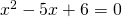which can be solved easily by factorization: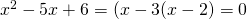and thus the solutions are: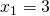or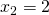We could also have applied the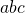-formula: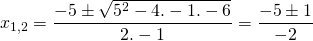and thus we get the same solutions.

0